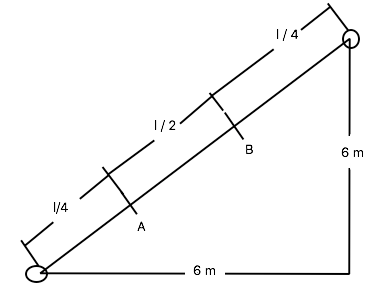# Two in-phase loudspeakers, which emit sound in all directions, are sitting side by side. One of...

## Question:

Two in-phase loudspeakers, which emit sound in all directions, are sitting side by side. One of them is moved sideways by 6.0 m, then forward by 6.0 m. Afterward, constructive interference is observed 1/4 and 3/4 of the distance between the speakers along the line that joins them. What is the maximum possible wavelength of the sound waves?

## Interference of Waves

Interference happened when two waves superpose to form a resultant wave, the resultant wave can be of greater, lower, or the same amplitude as the two reference wave. There are two kinds of interference, constructive and destructive interference which are the result of the interaction of waves. Constructive interference happened when the two waves add up to each other and destructive interference happened when two waves cancel each other.

The condition for constructive destructive interference is given by the following formula,

{eq}\Delta x = n \lambda \ ..... \ ( for \ constructive \ Interference ) \\ \Delta x = (n + \frac 12) \lambda \ ..... \ ( for \ destructive \ Interference ) \\ {/eq}

Wherein, n is an integer and

{eq}\lambda {/eq} is the wavelength of the wave.

Consider the figure,There is constructive interference in A and B, so

{eq}\Delta x_{max} {/eq} is between A and B = l/2

{eq}\Delta x = n \lambda {/eq} for constructive Interference,

{eq}n \lambda = \dfrac l2 \ ....... \ for \ max \ \lambda, \ n \ = \ 1 \ , \\ \\ \lambda = \dfrac l2 {/eq}

We need to calculate the distance "l" between the speakers,

{eq}l \ = \ \sqrt{6^2 + 6^2} \\ \ = \ 8.485 \ m\\ \\ \dfrac {8.485}{2} = n \times \lambda \\ for \ n = 1 \\ \lambda = 4.24 \ m \\ {/eq}

The maximum possible wavelength of the sound waves 4.24 meters.